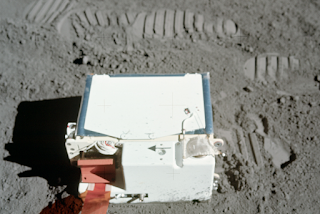## Saturday, February 8, 2020

### Atmospheric Drag

Suppose it is true that Apollo 10 Lunar Module descent stage, aka "Snoopy", has not decayed out of orbit due to lumpy lunar gravity. What other factors might have brought the stage down in the intervening 50 years? That's a long time, so even subtle effects could come into play, including the nearly non-existent lunar atmosphere.

The space around the moon is nearly a perfect vacuum, by Earthly standards. But "nearly perfect" means that there are still thousands of molecules in each cubic centimeter of space around the moon. In fact scientists refer to the lunar atmosphere as an "exosphere" because there are so few molecules that they rarely collide with each other. They behave more like an army of tiny satellites, moving in response to gravity or electrostatic forces.This instrument was placed on the moon's surface during Apollo 17 to study the moon's exosphere.

According to this source, there are around 155,000 molecules in each cubic centimeter of the lunar exosphere. Yikes! That seems like a very big number. When you think that the descent stage is 12 feet on a side, and moving at almost a mile a second, that is a LOT of collisions with lunar molecules. Then take that out 50 years...now it's REALLY a lot. If we calculate the mass of all those molecules, and compare that to the mass of the stage, we can get an idea about whether the exospheric drag might have brought down the stage. If the mass of the gas is even close to the mass of the stage, that would be a real drag.First of all lets estimate how many cubic centimeters the stage passes through every second. Just to get an upper bound on it, let's assume the stage is always moving with it's largest side facing forwards, sweeping through the largest area. We can approximate it as a square that is 12 feet on a side, which works out to an area of 140,000 square centimeters. (To get to this square I add the area of the foot pads into the missing corners of the stage, which is actually octagonal. Remember we are just estimating here.)

Now, the stage is moving about one mile every second. It moves faster when it drops down closer to the moon, and slower when it reaches it's "apolune" high point, but we can average that out and just assume a fixed velocity, as if it were in a circular orbit. One mile is 1.6 kilometers or 160,000 centimeters. Every second. Day after day. Month after month. Perhaps even decade after decade?

Well, now it seems like we might have a problem. If the stage is sweeping through an area of 140,000 square centimeters, and covering 160,000 centimeters per second, that works out to 22 billion cubic centimeters every second. And remember, if every cubic centimeter has 155,000 molecules, it means the stage is colliding with around 3.5 quadrillion molecules. Every second. Day after day. OK, then, perhaps all these collisions slowed down the stage, and it hit the moon after all?

Now we have to figure out how much all those gajillions of molecules weigh. Fortunately, remembering high school chemistry, this is easy. With a periodic table of elements, we take the atomic weight of each molecule, and then add them up. So for instance each Helium4 molecule has an atomic weight of 4 (or close enough for this estimate) so 40,000 of them total to 160,000 atomic units. Total up all the other molecules in the same way and it comes out to about 2.5 million atomic mass units.

OK, so now how much is that in units I can comprehend? It seems like a lot! Well, it turns out to be very little, because each molecule weighs very, very, very little. For instance one Helium atom, in grams, weighs about 0.00000000000000000000000166 grams. We better start using scientific notation, so that would be written as 1.66E-24. Now multiply that by the 2.5 million atomic mass units, and it come out to 4.13E-18 grams per cubic centimeter. Which is not much. (Yes, I am assuming the lunar exosphere is uniformly dense, which I know is not true. At this point I just want to get a feel for the problem.)

So now we have a war between big numbers and small numbers, that is, between the very large number of cubic centimeters (or cc's) that the stage sweeps through every second, 22 billion, versus the very small weight of the gas in each cc. So 22 billion times 4.13e-18 comes out to 9.2E-8 grams. Let's write that out as 0.000000092 grams. Every second. So far the small numbers are winning. That is the weight of the stuff the stage is running into every second, and it isn't much. And if we imagine each molecule as stationary when the stage strikes it, and moving away at the velocity of the stage after the collision, each collision will rob the stage of that tiny amount of momentum. (Think of a billiard ball striking another, stopping the first ball and sending the second one off with the momentum of the first.) So again, 9.2E-8 grams of collisions every second.

So now we just multiply by the number of seconds in 50 years. One year is 31.5 million seconds, and 50 years works out to 1.58E+9 seconds. Now the seconds help the big numbers to win the war, but not by much. The 9.2E-8 grams times 1.58E+9 seconds comes out to 146 grams. About one-third of a pound. Compare that to the weight of the stage. It's dry weight was 4700 pounds. So colliding with one-third pound of lunar exosphere over the course of 50 years could have slowed down the stage by 0.007%...i.e. nada!

So far, it seems that lumpy gravity didn't bring the stage down, and neither did atmospheric (or exospheric) drag. Perhaps Snoopy is still out there!

1.1.